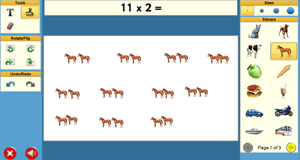# 2 x’s Tables

Classroom Resource

Learning Level: Elementary School

Learning Level: Primary SchoolThis gridset is designed to support students learning multiplication by 2.
In the Read about the concept gridset, the student is shown multiples of 2 through with pictures.
In the Show the concept gridset, the student makes his/her own book using Clicker Paint about the multiples of 2 that are indicated in print.
In the Errorless Practice in Sequence gridset, the student learns the 2 x’s table in sequence. 5 alternative answers are provided and only the correct answer is accepted.
In the Practice in Sequence gridset, the student practices the 2 x’s table in sequence, selecting the answer from 5 alternatives.
In the Mixed Practice gridset, the student practices multiples of 2 in mixed order, selecting the answer from 5 alternatives. This activity supports the Ministry of Education Learning Outcomes for Mathematics- demonstrate an understanding of multiplication to 5 × 5 by
– representing and explaining multiplication using equal grouping and arrays
– creating and solving problems in context that involve multiplication
– modelling multiplication using concrete and visual representations, and recording the process symbolically
– relating multiplication to repeated addition
– relating multiplication to division.
Created by Kathy Ryan.

Under BC’s new curriculum (2016):
Mathematics Grade 3: multiplication and division concepts
Mathematics Grade 4: multiplication and division facts to 100 (introductory computational strategies) = Students should be able to recall the following multiplication facts by the end of Grade 4 (2s, 5s, 10s).

## Resource Files

Please Note: These files can only be downloaded on desktop. Visit the SET-BC website on a desktop computer to access them.